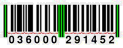## UPC Check Digit

The final digit of a Universal Product Code is a check digit computed so that summing the even-numbered digits, plus 3 times the odd-numbered digits, modulo 10, is 0.For example, take the UPC 070617006092. The sum of the even numbered digits is 7+6+7+0+0+2 = 22, and the sum of the odd-numbered digits is 0+0+1+0+6+9 = 16. The total sum is 22+3×16 = 70 ≡ 0 (mod 10). So the code is valid.

The input will contain five lines of data. Each line will contain a 12 digit UPC that may have an invalid check digit.

The output will contain five lines of data. Each line will contain the UPC with the correct check digit.

### Sample Input

```070617006093
036000291455
123456789097
246809753116
543210987665```

### Sample Output

```070617006092
036000291452
123456789098
246809753116
543210987667```

Point Value: 5
Time Limit: 1.00s
Memory Limit: 16M